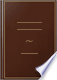Αναζήτηση Εικόνες Χάρτες Play YouTube Ειδήσεις Gmail Drive Περισσότερα »
Είσοδος
 Βιβλία Βιβλία 11 - 20 από 132 για Multiply the divisor, thus augmented, by the last figure of the root, and subtract....Multiply the divisor, thus augmented, by the last figure of the root, and subtract the product from the dividend, and to the remainder bring down the next period for a new dividend.The National Arithmetic, on the Inductive System: Combining the Analytic and ... - Σελίδα 27
των Benjamin Greenleaf - 1839 - 305 σελίδες
Πλήρης προβολή - Σχετικά με αυτό το βιβλίο## Arithmetic Modernised: Or, A Complete System of Arithmetic, Adapted to ...

John Davidson, Robert Scott (writing master) - 1818 - 172 σελίδες
...three parts will be the complete divisor, which multiply by the last figure of the root, subtract the product from the dividend, and to the remainder bring down the next part for a new dividend. Proceed in the same manner as before to find the divisor and the third figure...## A Complete System of Theoretical and Mercantile Arithmetic: Comprehending a ...

George G. Carey - 1818 - 574 σελίδες
...divisor; then multiply the divisor (thus increased) by the figure last put in the quotient, subtract the product from the dividend, and to the remainder, bring down the next period for a new dividend. Find the next figure of the root, as before, by doubling that part of the...## Temple's Arithmetick: A Concise Introduction to Practical Arithmetick: in ...

Samuel Temple - 1825 - 107 σελίδες
...dimtti being the denominator. This fractional part belongs to the quotient. her ; subtract as before, and to the remainder bring down the next figure of the dividend ; thus proceed, till every figure of the dividend is brought down. JVo/e. If, after a ijgure is brought...## The Youth's Assistant in Theoretick and Practical Arithmetick ...

Zadock Thompson - 1826 - 164 σελίδες
...right hand of the divisor ; multiply the divisor by the figure in the root last found, subtract the product from the dividend, and to the remainder bring down the next period fora new dividend. 4. Find a divisor as before, by doubling the figures in the root, and proceed...## The Common School Manual: A Regular and Connected Course of Elementary ...

...place (he product under that part of the dividend to which the divisor was applied, and subtract. 3. To the remainder, bring down the next figure of the dividend, and see how often the divisor will go, and place tfts in the quotient. 4, Then multiply and subtract as...## The Juvenile Arithmetick, and Scholar's Guide: Wherein Theory and Practice ...

Martin Ruter - 1828 - 166 σελίδες
...dividend before mentioned. Subtract this product from that part of the dividend under which it stands, and to the remainder bring down the next figure of the dividend; but if this will not contain the divisor, place a cypher in the quotient, and bring down another figure...## A Short System of Practical Arithmetic: Compiled from the Best Authorities ...

William Kinne - 1829
...or the next less than, the dividend, and it will be the second figure of the root. 5. Subtract that product from the dividend, and to the remainder bring down the next period for a new dividend. 6. Find a divisor as before, by doubling the figures already in the root...## Practical and Mental Arithmetic: On a New Plan, in which Mental Arithmetic ...

...whole increased divisor bit the last quotient figure, and write the product under the dividend. ' VI. Subtract this product from the dividend, and to the remainder bring down the next -period, for anew dividend. VII. Double the quotient figures, that is, the root already found, and...## The Tutor's Assistant: Being a Compendium of Arithmetic and a Complete ...

Francis Walkingame - 1832 - 208 σελίδες
...Line : but when the Divisor is more than 13, multiply it by the Quotient Figure, the Product subtract from the Dividend, and to the remainder bring down the next Figure in the Dividend, and proceed as before, till the Figures are all brought down. PROOF. Multiply the...## The Arithmetical Manual

Samuel Read Hall - 1832 - 288 σελίδες
...the right of the divisor. Multiply the divisor by the figure in the root last found ; subtract the product from the dividend, and to the remainder bring down the next period for a new dividend. Double the root now found for a divisor, and proceed as before to find the...